CiteScore 3.0
More on impact ›

# Frontiers in Mechanical Engineering## ORIGINAL RESEARCH article

Front. Mech. Eng., 28 January 2020 | https://doi.org/10.3389/fmech.2019.00074

# Adaptive Step Size Control of Extended/Unscented Kalman Filter Using Event Handling Concept

• Chair of Dynamics and Control, University of Duisburg-Essen, Duisburg, Germany

This paper presents a novel (Extended/Unscented) Kalman Filter by augmenting the event handling procedure of Ordinary Differential Equation (ODE) solvers with the predictor-corrector scheme of Extended/Unscented discrete Kalman Filter (EKF/UKF) introducing a variable step size Kalman Filter. This innovation allows a new quality of precision while operating Kalman Filters. The original Kalman Filter is based on a time-discrete predictor-corrector scheme considering fixed step size. The main new idea is to introduce and control step size handling not introduced before. Step size control of EKF/UKF will increase the performance when applied to switching/stiff systems. The step size is controlled based on the current performance (EKF/UKF innovation) and is adapted during the estimation procedure based on an event handling algorithm. The proposed event handling algorithm consists of two parts: relaxing sample time and restricting sample time (ST). Relaxing procedure is used to avoid high computational time when no rapid change exists in system dynamics. Restricting procedure is considered to improve the estimation performance by decreasing the EKF/UKF step size in the case of fast dynamical behavior (switching/stiff behavior). Effectiveness of the proposed approach is verified considering the well-known Van der Pol oscillator as a common example of stiff systems.

## 1. Introduction and Problem Definition

Step size control normally contains event handling procedure to detect the moment in time of specific event. Event handling and zero crossing detection are well-known and well established approaches to handle numerical simulation (Watts, 1984; Zhang et al., 2008). The idea of improving the performance by detecting the moment in time at which events take place is known in the field of ODE solvers. In Esposito and Kumar (2007) an event detection algorithm is introduced for non-smooth differential equations. In this paper an extrapolation polynomial is used for selection of the integration step size to detect potential future events. This approaches has advantages especially in the neighborhood of model singularities where the derivative function is undefined in state space. Singular systems can be modeled as Markovian jump singular systems. Investigation of stability, stabilization, control, and filtering of these systems are more complicated compared to the systems modeled in state space (Wang et al., 2017).

In Bernal (1991) the author addressed calculation of time-step sizes to prevent interstep events in multilinear problems. In this work an approach is introduced to locate the moment in time when the stiffness changes in a non-linear system. This approach can be considered as an interpolation scheme of the time when events occur by using computed responses at the end of corresponding linear step. In Wright and Pei (2012) an explicit numerical method is proposed to solve differential algebraic equations (DAE) considering low-order discontinuities in the restoring force algebraic equations. The effectiveness of this approach is verified for a specific class of problems namely non-smooth DAE with discontinuities. The algorithm is able to automatically detect each state event location.

In reality the system dynamics is modeled by linear or non-linear ordinary differential equations. For example in Bakhshande and Söffker (2015) an elastic beam is modeled as a linear ODE. A high gain Proportional-Integral-Observer (PIO) introduced in Söffker et al. (1995) is used to estimate the node displacements of the elastic beam system as well as the disturbance force affects the system dynamics. The dynamics of system and observer are augmented and described in one linear ODE form. The aforementioned example can be described by a suitable initial value problem (IVP). In this case stiff solvers able to handle stiff problems are used to avoid high computational time by relaxing the integration step size when no rapid change exists in system states and disturbance dynamics, as well as to improve the estimation results by decreasing the integration step size in the case of fast dynamical behavior related to disturbances (stiff behavior). In Bakhshande and Söffker (2015) simulation results are achieved using stiff ODE solver in Matlab (ode15s) considering event handling and zero crossing procedures.

Kalman Filter and its extensions (EKF and UKF) has the same functionality as observers for discontinuous systems and measurements containing statistical noise and other inaccuracies. Different approaches have been introduced to improve the performance of KF and EKF/UKF. For example iterated extended Kalman filter (IEKF) (Bell and Cathey, 1993) linearizes the system non-linear equations iteratively to compensate the significant non-linearities. In this algorithm an iterative procedure is considered for measurements update (correction part). The iterative procedure is stopped if the maximum number of iterations is reached or the difference between two iterations results is less than a pre-specified threshold. In Skoglund et al. (2015) by assuming the EKF as an optimization problem, different optimization algorithms (e.g., Line Search, Quasi-Newton, and Levenberg-Marquardt) are considered to improve the performance and robustness of estimation. In Havlík and Straka (2015) an iterated extended Kalman filter with variable step-length is introduced. In this approach the sample time is considered as a fixed value. The measurement update step is calculated based on the Gauss-Newton algorithm or Line Search optimization procedure with variable step length. Here the step size is considered as step size of optimization procedure to ensure the convergence of a predefined criteria.

In Wang and Li (2008) an event-based Kalman Filter observer is proposed to control the angular position of a rotary high speed on/off valve. Measurements obtained at irregular time instants and detected by an encoder. The uncertainty on transition event occurrence time is considered as zero mean random measurement noise acting on rotary position. This measurement noise affected by the sampling time, is considered as part of the system model to be used for Kalman Filter estimation. In Chatzis and Chatzi (2017) a discontinuous Unscented Kalman Filter (DUKF) is proposed as an modified version of the UKF. This approach adapts the state space formulation, evaluates the observability within each time step, and selects the suitable sub-space that can be used by UKF. Consequently the unidentifiable parameters (related to non-smooth behaviors) are detected and excluded from the problem formulation.

An event triggered continuous discrete Kalman Filter is introduced in Niu et al. (2018). The MSE of estimation is controlled by detecting the event and taking a new measurement. This approach decreases the sample time at each step to reach the desired estimation error at the current sample time. The next estimation starts by reinitializing the sample time to the original one.

In all aforementioned approaches the sample time of estimation results is defined constant (based on the measurements) or is just reduced at the current step and is reinitialized for the next time step. The goal is to achieve a suitable estimation performance (in the case of event). None of the mentioned approaches focus of sample time control considering event detection by increasing and reducing the sample time during the estimation procedure (like ODE solvers). In this contribution the sample time is controlled based on the estimation performance. It decreases in the case that estimation error increases to achieve the desired performance. Correspondingly it increases when the performance is suitable enough to reduce the computational effort.

Event handling and zero crossing procedures have been used and improved over the previous years for solving stiff ODEs. The main objective of this paper is to integrate the benefits of event handling and zero crossing procedures into the discrete predictor-corrector scheme of Extended/Unscented Kalman Filter to adaptively control the step size. Procedure of KF starts from an initial set of Kalman parameters and system states. The next estimation step is realized based on the current step information (same concept as IVP). Generally the predictor-corrector approaches are based on a pre-specified tolerance. To use the KF for switching/stiff problems the algorithm should be able to look back, execute the solution with smaller step size (stiff problems), execute the solution at precisely defined moments in time (switching problems), and reach the predefined tolerance. Therefore, in this contribution a novel Extended/Unscented Kalman filter is proposed by augmenting the variable step size control in the predictor-corrector scheme for the stiff/switching systems. The proposed variable step size Extended/Unscented Kalman Filter is able to solve the state estimation of stiff problems in discrete time to avoid unnecessary high computational load if required and to increase estimation performance. Using the proposed variable step size Extended/Unscented Kalman Filter allows step size control inside the predictor-corrector procedure. Sample time is adapted based on current estimation performance (EKF/UKF innovation) of system states.

This contribution is based on the previously published initial research paper (Bakhshande and Söffker, 2018). In Bakhshande and Söffker (2018) the first idea is presented for linear systems and correspondingly Kalman Filter. Extending and detailing the initial work, in this contribution an extended and improved version of step size control is proposed for non-linear dynamical behavior considering EKF and UKF. Furthermore, adjustment of design parameters is investigated in this contribution to get a suitable convergence rate and estimation error.

Unscented Kalman Filter is used here as a non-linear discrete filter which can be replaced by any other discrete filter for linear or non-linear systems. The main contributions of this paper can be summarized as follows:

• Introducing a novel event-triggered discrete EKF/UKF for stiff/switching non-linear systems based on the event handling procedure in ODE solvers.

• Adaptation of time step in EKF/UKF (discrete world) based on the Kalman innovation as key contribution of this paper (step size control in discrete world).

• Controlling the innovation of estimation error for EKF/UKF and computational effort under designable thresholds (α and β).

The paper is organized as follows: First a general definition of stiff/switching systems is provided besides the common solutions to solve stiff ODEs. In the second part main aspects of (Matlab-based) ODE solvers are introduced e.g., zero crossing and event handling procedures with the purpose of integration into the structure of Extended/Unscented Kalman Filter. Subsequently, the proposed variable step size Extended/Unscented Kalman Filter is detailed considering the structure and procedure of well-known discrete EKF/UKF. Furthermore, the proposed method is evaluated using simulation results of the Van der Pol oscillator (common example of switching/stiff system). The last section finalizes the paper with a summary and conclusions.

## 2. Stiff/Switching System

Ordinary Differential Equation (ODE) contains derivative(s) of a dependent variable (usually denoting as x and corresponding derivative(s) as , , …) with respect to a single independent variable (usually referring to time t). The initial Value Problems (IVP) are iteratively solved by assuming an initial condition x(t0) = x0 and the time period of interest t = [t0, tf]. At each step the solver uses the results of previous step considering a particular algorithm (Euler's method, RungeKutta methods, etc.). The final result is given as a vector of time steps t = [t0, t1, …, tf] and corresponding sequence of values for the dependent variable x = [x0, x1, …, xf]. Higher-order differential equations can be reformulated at each step of iterative solving procedure of IVP as a system of first-order equations:

with step size:

and corresponding solution:

Generally even if function f is a continuous function there is no guarantee that the IVP provides a unique solution. Considering Picard's theorem according to Süli (2001) and considering Lipschitz condition for function f, it can be stated that a unique solution exists for Equation (1).

In Atkinson et al. (2011) a linear approximation of the general ODE (Equation 1) is introduced as:

with A = fx(t0, x0) as m × m Jacobian matrix of f evaluated at (t0, x0).

Definition 1. [According to Hairer and Wanner (1987)]: The system ẋ(t) = f(t, x(t)) is called separably stiff at a position (t0, x0) if the Jacobian $J=\frac{\partial f}{\partial x}\left({t}_{0},{x}_{0}\right)$ possesses k < n eigenvalues λ1, λ2, …, λk such that,

The eigenvalues λ1, λ2, …, λk are called the stiff eigenvalues and,

the relative separation.

Stiff/switching system equations contain some terms that produce a fast variation in the solution. According to Hairer and Wanner (1987) relative separation can define the stiffness level. In other words, the eigenvalue responsible for the slowest rates of change should be compared to those leading to the fastest rates of change.

Considering non-linear systems the complexity increases because in this case stiffness becomes a global property and therefore cannot be reduced to a local problem by using a solution in the neighborhood of single points. The non-linear problem may start with non-stiff behavior and become stiff, or vice versa. It may contain stiff and non-stiff intervals. In Ashino et al. (2000) a comprehensive definition of stiff system is introduced for linear and non-liner systems as the following.

Definition 2. If a numerical method is forced to use, in a certain interval of integration, a step length which is excessively small in relation to the smoothness of the exact solution in that interval, then the problem is said to be stiff in that interval.

To solve the numerical integration of stiff ordinary differential (Equation 1) a suitable small step size hn is required in the case of fast variation in the solution and correspondingly the step size has to be relatively large (relaxed) when the solution is smooth. Consequently a perfect numerical solution is able to solve the stiff and non-stiff ODEs denoted as step size control (Watts, 1984). Stiff numerical methods have the ability to change the step size during solving procedure. They take small steps to obtain satisfactory results nearby solutions that vary rapidly. The main advantage of stiff solvers is the low computational time compared to non-stiff solvers. Non-stiff solutions can be used for stiff problems with a proper small step size but it takes more time to achieve the final solution because the step size is constant and can not be adapted according to the actual results.

## 3. Zero Crossing and Event Handling Procedures

In this section the main ideas of zero crossing and event handling are repeated to be used later introducing the proposed algorithm. Here they are briefly repeated because exactly these ideas will be transferred to the finite difference scheme of calculation digital filters like Kalman Filter.

As discussed in section 1, variable step size solvers increases or decreases the step size to achieve error tolerances and required or given performance. Selection of fixed or variable step size depends on the dynamical model and implementation issues. The fixed step size solver uses one step size for the whole simulation time and consequently the step size has to be small enough to achieve the accuracy requirements.

Implicit variable step size solvers (stiff solvers) can be used to solve stiff problems. The non-stiff solvers are ineffective on intervals where the solution changes slowly because they use time steps small enough to resolve the fastest possible change for the whole estimation time. Furthermore, the step size of non-stiff solvers has to be defined at the initialization level and can not be changed during the solving procedure.

Event handling and zero crossing procedures are usually considered in ODE solvers. Detecting the moment in time of specific events in ODEs is referred to event handling procedure. For example the exact time a ball hits the ground or the time that the ODE solutions reaches a specific value is a very specific moment. The event is detected from time step tn to tn+1 if the conditional statement (event) becomes true. The zero crossing detection algorithm is a procedure to capture and locate events accurately. In the case of zero crossing for a predefined event, the ODE solver can be terminated or continued with different conditions according to demand. Event detection of ODE solvers contains two functions f(t, x) and g(t, x), and an initial condition (t0, x0). Using event handling procedure in the structure of ODE solver means to numerically define the moment in time t* so that:

Here g(t, x) denotes the event condition which has to be defined by the programmer according to the requirements.

## 4. Extended/Unscented Kalman Filter

Filtering is an important and fundamental problem in the discrete-time control field. For example in Wang et al. (2019) a fuzzy filter is designed for discrete-time fuzzy descriptor systems considering the limited unreliable communication links. One of the most important filter structures in the field of control is Kalman Filter. Principally, Kalman Filter can be used (i) for system state estimation when it can not be directly measured or (ii) for fusion of sensors information when measurements are available from various sensors but might be subject to noise. Although many applications can be considered for this filter, these are two of the most important tasks and applications of this filter.

The main goal of this filter is to achieve final estimation with zero bias and minimum variance compared to the real value and considering noisy measurements. Kalman filter is the optimal linear filter in sense of minimizing the variance estimate of states with the assumptions that the system model perfectly matches the real system, the entering noise is white (uncorrelated), and the covariances of the noise are exactly known (Anderson and Moore, 2012). In other words, for systems fulfilling the requirements, KF is the best choice. Kalman Filter is firstly introduced in Kalman (1960) to formulate and solve the Wiener Filter problem which is proper for filtering, smoothing, and prediction of wide-sense stationary signals. It is worth noting that Kalman Filter in discrete or continuous form and its extensions (Bishop and Welch, 2001) are introduced as a fundamental algorithm to solve a broad range of estimation problems.

Extended Kalman Filter (EKF) has been introduced for non-linear systems by linearizing the estimation around the current estimation and using partial derivatives of the system/measurement equation (Sorenson, 1960; Costa, 1994). In the presence of strong non-linearities, Extended Kalman filter leads to poor estimation results because of propagation of covariance from one measurement sample time to the next through the linearization (Bishop and Welch, 2001). In other words using of Jacobians, representing all partial derivatives of the non-linear system (model), may lead to sub-optimal performance and sometimes divergence of the extended Kalman Filter. Therefore, Unscented Kalman Filter (UKF) is introduced in Costa (1994) to improve the estimation performance as well as to remove the requirement of Jacobians calculations. The UKF exactly addresses the problem of EKF by using a deterministic sampling approach. The UKF approximates the state distribution by a GRV (Gaussian Random Variable) as well as EKF, but it is represented by using a minimal set of carefully chosen sample points.

The Extended Kalman Filter assumes that the state at time step k is calculated from the previous state step xk−1 according to:

In a general system either the state transition function f or the measurement function h or both may be non-linear. Here the process and measurement noises (wk and vk) enter the system linearly but there may be systems for which the noise can not be assumed as additive. They are assumed to be zero mean Gaussian noises with covariance Q and R respectively. In the procedure of EKF the non-linear functions are linearized around the mean of current state estimation as:

At each time step the linearization is performed locally and the resulting Jacobian matrices JF and JH are then used in the prediction and correction steps of the KF algorithm. The system and measurement noise respectively wk and vk are assumed to be independent, white, and with normal probability distributions as:

In practice, the noise covariance matrices might change during the time, however they are often assumed to be constant. There are two sets of equations for prediction and correction process. The time update projects the current state estimation ahead in time while the measurement update adjusts the projected estimation by an actual measurement at that time. The procedure of Extended Kalman Filter can be summarized as follows:

Prediction (time update)

${\stackrel{^}{x}}_{k}^{-}=f\left({\stackrel{^}{x}}_{k-1},{u}_{k}\right)$

(2) Project the error covariance ahead

${P}_{k}^{-}={J}_{F}{P}_{k-1}{J}_{F}^{T}+Q$

Correction (measurement update)

(1) Compute the Kalman gain

${K}_{k}={P}_{k}^{-}{J}_{H}^{T}{\left({J}_{H}{P}_{k}^{-}{J}_{H}^{T}+R\right)}^{-1}$

(2) Update the estimation with measurement

${\stackrel{^}{x}}_{k}={\stackrel{^}{x}}_{k}^{-}+{K}_{k}\left({z}_{k}-h{\stackrel{^}{x}}_{k}^{-}\right)$

(3) Update the error covariance

${P}_{k}=\left(I-{K}_{k}{J}_{H}\right){P}_{k}^{-}$

When the system is non-linear and can be well approximated by linearization then the EKF is a good option for state estimation. However it has the following drawbacks:

1. It may be difficult to calculate the Jacobians if they need to be calculated numerically.

2. There are high computational costs when the Jacobians are calculated numerically.

3. The EKF only works on systems that have a differentiable model.

4. The EKF is not optimal if the system is highly non-linear (linearization does not provide a good approximation for highly non-linear systems).

Unscented Kalman Filter (UKF) can be used to overcome the last disadvantage of the EKF by approximating the probability distribution instead of approximating the non-linear function. A brief comparison of KF, EKF, and UKF is presented in Table 1 considering the main principles of filtering. The computational cost of Kalman Filter depends also on the sample time of the measurements which is the main focus of this paper.

TABLE 1

## 5. Variable Step Size Extended/Unscented Kalman Filter

In section 4 the procedure of standard Extended/Unscented Kalman Filter and correspondingly predictor-corrector scheme has been briefly explained. In the following section variable step size (Extended/Unscented) Kalman Filter is proposed and discussed in detail to enhance the performance of (Extended/Unscented) Kalman Filter for stiff/switching problems. The proposed approach consists of event handling and zero crossing concepts according to ODE solvers with variable step size integration. The main flowchart of variable step size Kalman Filter is graphically and mathematically defined in Figure 1 and Algorithm (1).

FIGURE 1
ALGORITHM 1

The algorithm starts by initialization of Kalman Filter prerequisites e.g., initial states, covariance matrices, and initial sample time (ST). At the first step (initialization level) or in the case that an event is occurred and detected during the solving procedure (ST is decreased or increased) the state space model of the system is discretized based on the new ST. Since the measurement vector is constant, interpolation is required to define the measurement vector according to new sample time. The Kalman Filter procedure is solved from the zero moment up to the current time to find a suitable initial value for the upcoming time. At each step of predictor-corrector scheme the Kalman Filter innovation is used for event handling procedure (shown in detail in Figure 1). The proposed event handling algorithm consists of two main parts: restricting ST and relaxing ST. Four parameters are considered as design parameters (Min. ST, Max. ST, α, and β). The α and β parameters denote the upper and lower thresholds of accuracy and are defining the compromise between computational time and estimation accuracy. The Min. ST and Max. ST are defined to show the maximum and minimum possible sample time that should be defined according to the desired performance.

When ST changes the predictor-corrector scheme is stopped. According to Figure 1 the variable step size Kalman Filter procedure is continued by applying a new discretization of system model and new initialization using interpolated measurement vector. The procedure is continued until the final time of simulation and at each sample k the results are stocked to be released at the end of simulation time.

## 6. Non-linear Example: Van der Pol Oscillator

To verify the proposed approach, namely variable step size EKF/UKF, the Van der Pol oscillator example is considered. The Van der Pol oscillator is a highly non-linear system/oscillator with non-linear damping. It can be considered as non-stiff or stiff example considering the strength of damping. This system is used in literature as a switching example to show the advantages of event handling and variable step size ODE solvers (Khan et al., 2011). Therefore, it is used here to illustrate the advantages of proposed variable step size EKF/UKF including event handling procedure. The dynamics of Van der Pol oscillator evolves in time according to the following second-order differential equation:

where x denotes the position coordinate as a function of time and μ is a scalar parameter representing the non-linearity and strength of damping. The equations become increasingly stiff as μ increases and for μ = 0 the system becomes linear. The system (Equation 11) can be rewritten in state space form as:

with state vector ${\left[{x}_{1}{x}_{2}\right]}^{T}$ describing the first and second states of the Van der Pol system. Accordingly a first-order Euler discretization of the equations of motion of Van der Pol oscillator is calculated as:

with,

where h denotes the assumed sample time for discretization procedure.

The Van der Pol oscillator is driven by the system and measurement noise according to Equation (8) with zero mean white Gaussian noise for system and measurement with covariances Q in R2×2 and R > 0. In this paper it is assumed that for k ≥ 0 both states are available as measurements. The objective is to obtain the estimation ${\stackrel{^}{x}}_{k}$ using the measurements considering different approaches namely EKF, UKF, VSEKF, and VSUKF and to compare the estimation performance.

## 7. Numerical Results and Discussion

To investigate the performance of proposed improved EKF/UKF using the variable step size algorithm, introduced in this paper, simulation results were carried out using the Van der Pol oscillator system. The system parameters used in this simulation study, the KF covariances, and the noise parameters are considered identical for all simulations. Simulation results are illustrated as estimation of x1 in Figure 2, estimation of x2 in Figure 3, and respectively the phase portrait in Figure 4 for EKF and VSEKF. The performance is measured based on estimated states and related comparison with real ones. From the results it can be concluded that using the variable step size algorithm in combination with EKF produces better performance in estimation task especially nearby rapid behavior of the system (stiff behavior). In other words, the proposed solution improves the estimation results by decreasing the step size in the procedure of predictor-corrector scheme and in the case of fast dynamical behavior (time ∈ [0.2, 0.4]). On the other side by relaxing the step size when no rapid change exists, the proposed approach decreases the high computational time of predictor-corrector scheme (time ∈ [0.6, 1.6]).

FIGURE 2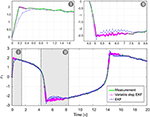Figure 2. Estimation of Van der Pol oscillator first state x1 using fixed and variable step size Extended Kalman Filter.

FIGURE 3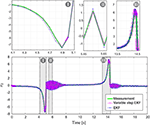Figure 3. Estimation of Van der Pol oscillator second state x2 using fixed and variable step size Extended Kalman Filter.

FIGURE 4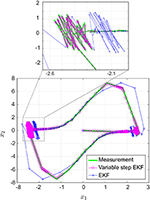Figure 4. Phase portrait illustration of Van der Pol oscillator using fixed and variable step size Extended Kalman Filter.

In Figure 5 the estimation performance (estimation error of the first system state namely $e={x}_{1}-{\stackrel{^}{x}}_{1}$) is illustrated for EKF, UKF, VSEKF, and VSUKF. In the right figure the results are achieved considering that the sample time is known for EKF and UKF while the left figure is the results related to unknown sample time. From the results it can be stated that even if the sample time is known for the procedure of EKF and UKF, the proposed VSEKF and VSUKF reach better estimation performance namely almost zero median error and improved minimum and maximum error values. The estimation results are summarized in Table 2. It is evident from the numerical results that using the proposed variable step size algorithm leads to less RMSE and MAE for VSEKF and VSUKF.

FIGURE 5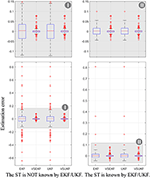Figure 5. Estimation error of Van der Pol oscillator state x1 using fixed and variable step size Extended/Unscented Kalman Filter.

TABLE 2

In the next simulation part the measurement and system noise variance is changed and the RMSE is calculated for all filters. The results are illustrated in Figure 6 with logarithmic axis for noise variance. From the results it can be concluded that the proposed approach produces more robust estimation results especially in the presence of measurement noise. The RMSE increases for EKF and UKF by increasing the noise variance while it has more or less no influence on the estimation results of VSEKF and VSUKF. As conclusion it can be stated that the proposed approach outperforms up-to-date solutions with respect to the different dimensions worth to consider while working with non-linear and or stiff systems.

FIGURE 6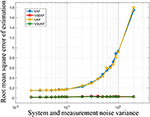Figure 6. Root mean square error (RMSE) of EKF/UKF and VSEKF/VSUKF considering different level of system and measurement noise.

## 8. Convergence Rate and Parameter Selection of VSEKF/VSUKF

As mentioned in section 5 the design parameters α and β are defined as upper and lower thresholds to control the innovation of estimation error and the computational effort correspondingly. In Figure 7 RMSE of the proposed approach is shown for variation of design thresholds α and β. In Figure 7A the total estimation time is considered while in Figure 7B the estimation error in the first 3 s is shown to evaluate the convergence rate of the proposed approach. From the results it can be concluded that the upper threshold α has the main influence on the estimation performance and by increasing the value of α the performance decreases. Furthermore, it can be concluded that increment of β threshold has less influence on the estimation error and convergence rate. The worse performance is related to the case that both thresholds are increased (as expected).

FIGURE 7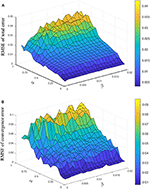Figure 7. Root mean square error (RMSE) of VSEKF considering different parameters α and β, (A) RMSE of total error of the estimation, (B) RMSE of convergence error in the first 3 s.

Next analysis is related to the influence of restricting/relaxing parameter d (which is considered to change the step size) on the estimation performance. According to Figure 8 the RMSE generally decreases by increasing the d parameter and it increases when d > 1.

FIGURE 8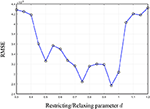Figure 8. Root mean square error (RMSE) of VSEKF considering variation of parameters d (Restricting/Relaxing parameter).

In Figure 9 the influence of system and measurement noise covariances on the estimation performance is illustrated. According to the result the performance decreases for system noise covariance 0 < Q < 100 and measurement noise covariance 250 < R < 800 (this area is illustrated with red arrows in Figure 9).

FIGURE 9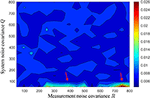Figure 9. Root mean square error (RMSE) of VSEKF considering variation of system and measurement noise covariances.

## 9. Summary, Conclusions, and Outlook

Variable step size (Extended/Unscented) Kalman Filter algorithm (VSEKF/VSUKF) proposed in this paper is based on event handling and zero crossing concepts involved in the structure of ordinary differential equation solvers. At each step of predictor-corrector scheme the Extended/Unscented Kalman Filter innovation is used to detect the events considering the predefined low and upper boundaries (α and β). In the case that an event is occurred and detected, the sample time is increased (relaxing procedure) or decreased (restricting procedure), the predictor-corrector scheme is stopped, and the procedure is continued by new discretization of system model and new initialization using interpolated measurement vector. In the case that no event is detected, the predictor-corrector scheme can be continued with the current considered ST. The design parameters α and β are considered to control and influence the performance and computational time.

The Van der Pol example is used as a common example for stiff systems to illustrate advantages of the proposed approach compared to standard discrete Exteneded/Unscented Kalman Filter. Simulation results clearly demonstrate the effectiveness of proposed estimation approach especially close to the rapid changes in the system dynamics (stiff behavior). In other words, the proposed solution improves the estimation performance by decreasing the step size in the procedure of predictor-corrector scheme according to current estimation accuracy and in the case of fast dynamical behavior. From the simulation results it can also be concluded that the proposed variable step EKF/UKF is more robust in the presence of high level of system/measurement noise compared to the standard EKF/UKF.

What is not covered and can be possibly detected as limitation of this work is the computational effort. Investigation of the computational effort related to the proposed step size control and its consequences are not considered in this contribution. The computational time needs for the proposed approach is more than standard EKF/UKF by reducing the step size. On the other side, in the relaxing procedure, computational time decreases. Therefore, investigation of parameter and thresholds definition regarding real-time implementation seems to be useful and can be considered as future work.

## Data Availability Statement

The raw data supporting the conclusions of this article will be made available by the authors, without undue reservation, to any qualified researcher.

## Author Contributions

FB and DS conceived of the presented idea developed the theory, performed the computations, and verified the analytical methods. All authors discussed the results and contributed to the final manuscript.

## Conflict of Interest

The authors declare that the research was conducted in the absence of any commercial or financial relationships that could be construed as a potential conflict of interest.

## Acknowledgments

We acknowledge support by the Open Access Publication Fund of the University of Duisburg-Essen.

## References

Anderson, B., and Moore, J. (2012). “Optimal Filtering,” in Dover Books on Electrical Engineering, ed T. Kailath (Dover Publications; University of Newcastle).

Ashino, R., Nagase, M., and Vaillancourt, R. (2000). Behind and beyond the matlab ode suite. Comput. Math. Appl. 40, 491–512. doi: 10.1016/S0898-1221(00)00175-9

Atkinson, K., Han, W., and Stewart, D. (2011). Numerical Solution of Ordinary Differential Equations. New York, NY: John Wiley & Sons.

Bakhshande, F., and Söffker, D. (2015). “Reconstruction of nonlinear characteristics by means of advanced observer design approaches,” in ASME 2015 Dynamic Systems and Control Conference, 2:V002T23A007. doi: 10.1115/DSCC2015-9897

Bakhshande, F., and Söffker, D. (2018). “Variable step size Kalman Filter using event handling algorithm for switching systems,” in ASME 2018 International Design Engineering Technical Conferences and Computers and Information in Engineering Conference (Quebec, QC) 6:V006T09A013.

Bell, B. M., and Cathey, F. W. (1993). The iterated Kalman filter update as a Gauss-Newton method. IEEE Trans. Autom. Control 38, 294–297.

Bernal, D. (1991). Locating events in step-by-step integration of the equations of motion. J. Struct. Eng. 117, 530–545.

Bishop, G., and Welch, G. (2001). An Introduction to the Kalman Filter. Department of Computer Science, University of North Carolina at Chapel Hill. Available online at: http://www.cs.unc.edu/~welch/kalman/kalmanIntro.html

Chatzis, M. N., and Chatzi, E. N. (2017). A discontinuous unscented kalman filter for nonsmooth dynamic problems. Front. Built Environ. 3:56. doi: 10.3389/fbuil.2017.00056

Costa, P. J. (1994). Adaptive model architecture and extended Kalman-Bucy filters. IEEE Trans. Aerospace Electr. Syst. 30, 525–533. doi: 10.1109/7.272275

Esposito, J. M., and Kumar, V. (2007). A state event detection algorithm for numerically simulating hybrid systems with model singularities. ACM Trans. Model. Comput. Simulat. 17:1. doi: 10.1145/1189756.1189757

Hairer, E., and Wanner, G. (1987). Solving Ordinary Differential Equations II. New York, NY: Springer.

Havlík, J., and Straka, O. (2015). Performance evaluation of iterated extended Kalman filter with variable step-length. J. Phys. Conference Series 659:12022. doi: 10.1088/1742-6596/659/1/012022

Kalman, R. E. (1960). A new approach to linear filtering and prediction problems. J. Fluids Eng. 82, 35–45.

Khan, J. A., Qureshi, I. M., and Raja, M. A. Z. (2011). Hybrid evolutionary computational approach: application to Van der Pol oscillator. Int. J. Phys. Sci. 6, 7247–7261. doi: 10.5897/IJPS11.922

Niu, Y., Cong, Y., and Wu, L. (2018). Event triggered continuous discrete kalman filter with controllable estimation error. IEEE Access 6, 42482–42496. doi: 10.1109/ACCESS.2018.2860626

Skoglund, M. A., Hendeby, G., and Axehill, D. (2015). “Extended Kalman filter modifications based on an optimization view point,” in 2015 18th International Conference on Information Fusion (Washington, DC), 1856–1861.

Söffker, D., Yu, T. J., and Müller, P. C. (1995). State estimation of dynamical systems with nonlinearities by using Proportional-Integral-Observer. Int. J. Syst. Sci. 26, 1571–1582.

Sorenson, H. W. (1960). Kalman Filtering: Theory and Application. New York, NY: IEEE Press.

Süli, E. (2001). Numerical Solution of Ordinary Differential Equations. Mathematical Institute, University of Oxford, Lecture note.

Wang, M., and Li, P. Y. (2008). “Event based kalman filter observer for rotary high speed on/off valve,” in 2008 American Control Conference (Seattle, WA: IEEE), 1546–1551. doi: 10.1109/ACC.2008.4586711

Wang, Y., Karimi, H. R., Lam, H.-K., and Yan, H. (2019). Fuzzy output tracking control and filtering for nonlinear discrete-time descriptor systems under unreliable communication links. IEEE Trans. Cybernet. 1–11. doi: 10.1109/TCYB.2019.2920709

Wang, Y., Xia, Y., Shen, H., and Zhou, P. (2017). Smc design for robust stabilization of nonlinear markovian jump singular systems. IEEE Trans. Automat. Control 63, 219–224. doi: 10.1109/TAC.2017.2720970

Watts, H. (1984). Step Size Control in Ode Solvers. Technical report, Sandia National Labs., Albuquerque, NM, United States.

Wright, J. P., and Pei, J.-S. (2012). Solving dynamical systems involving piecewise restoring force using state event location. J. Eng. Mech. 138, 997–1020. doi: 10.1061/(ASCE)EM.1943-7889.0000404

Zhang, F., Yeddanapudi, M., and Mosterman, P. J. (2008). Zero-crossing location and detection algorithms for hybrid system simulation. IFAC Proc. Vol. 41, 7967–7972. doi: 10.3182/20080706-5-KR-1001.01346

Keywords: Kalman Filter, extended Kalman filter, step size control, event handling, switching systems, stiff ODEs, Van der Pol system, zero crossing

Citation: Bakhshande F and Söffker D (2020) Adaptive Step Size Control of Extended/Unscented Kalman Filter Using Event Handling Concept. Front. Mech. Eng. 5:74. doi: 10.3389/fmech.2019.00074

Received: 13 September 2019; Accepted: 31 December 2019;
Published: 28 January 2020.

Edited by:

Hamid Reza Karimi, Politecnico di Milano, Italy

Reviewed by:

Eleni N. Chatzi, ETH Zürich, Switzerland
Mingyang Xie, Nanjing University of Aeronautics and Astronautics, China
Yueying Wang, Shanghai University of Engineering Sciences, China
Baoping Jiang, Suzhou University of Science and Technology, China

Copyright © 2020 Bakhshande and Söffker. This is an open-access article distributed under the terms of the Creative Commons Attribution License (CC BY). The use, distribution or reproduction in other forums is permitted, provided the original author(s) and the copyright owner(s) are credited and that the original publication in this journal is cited, in accordance with accepted academic practice. No use, distribution or reproduction is permitted which does not comply with these terms.

*Correspondence: Fateme Bakhshande, fateme.bakhshande@uni-due.de